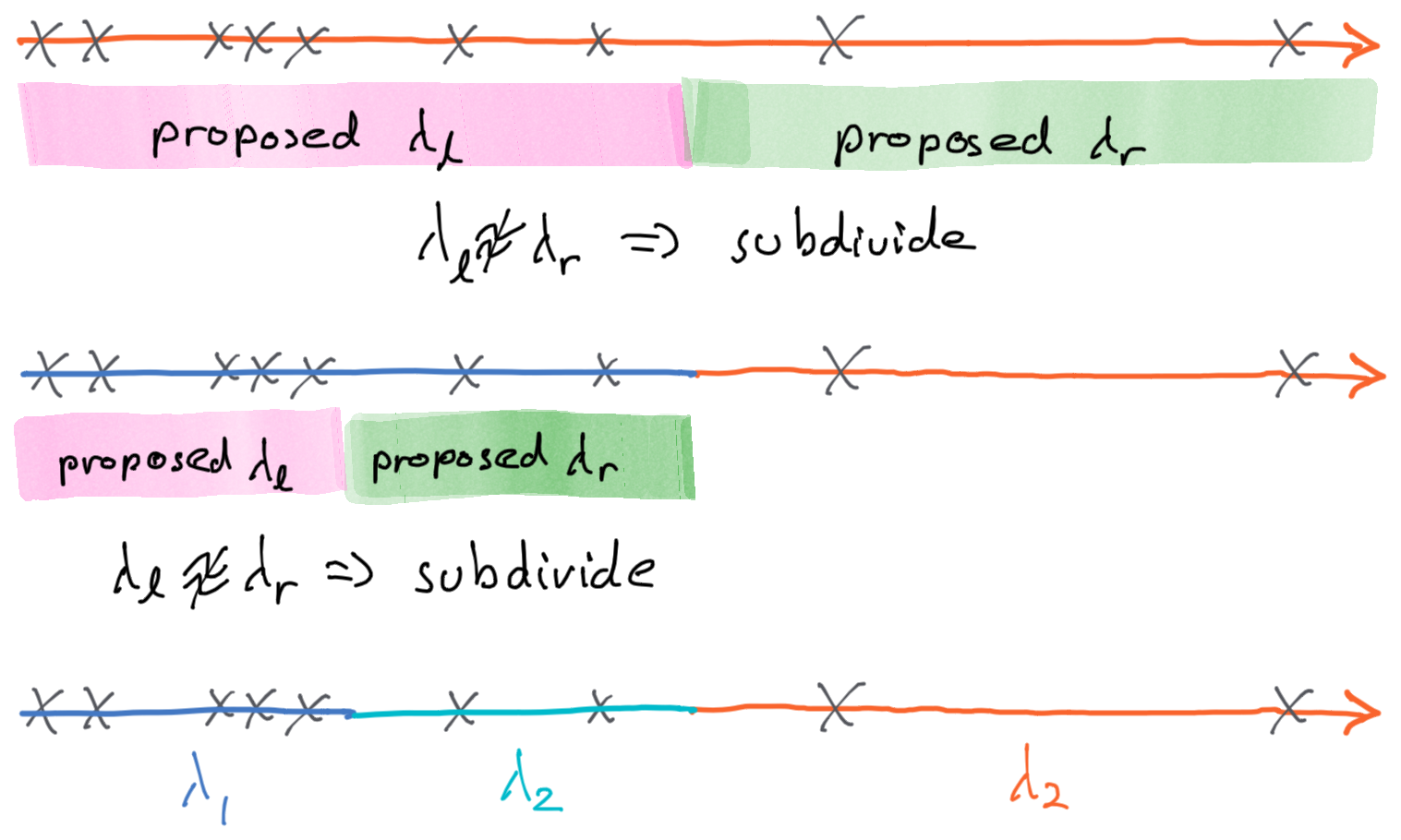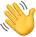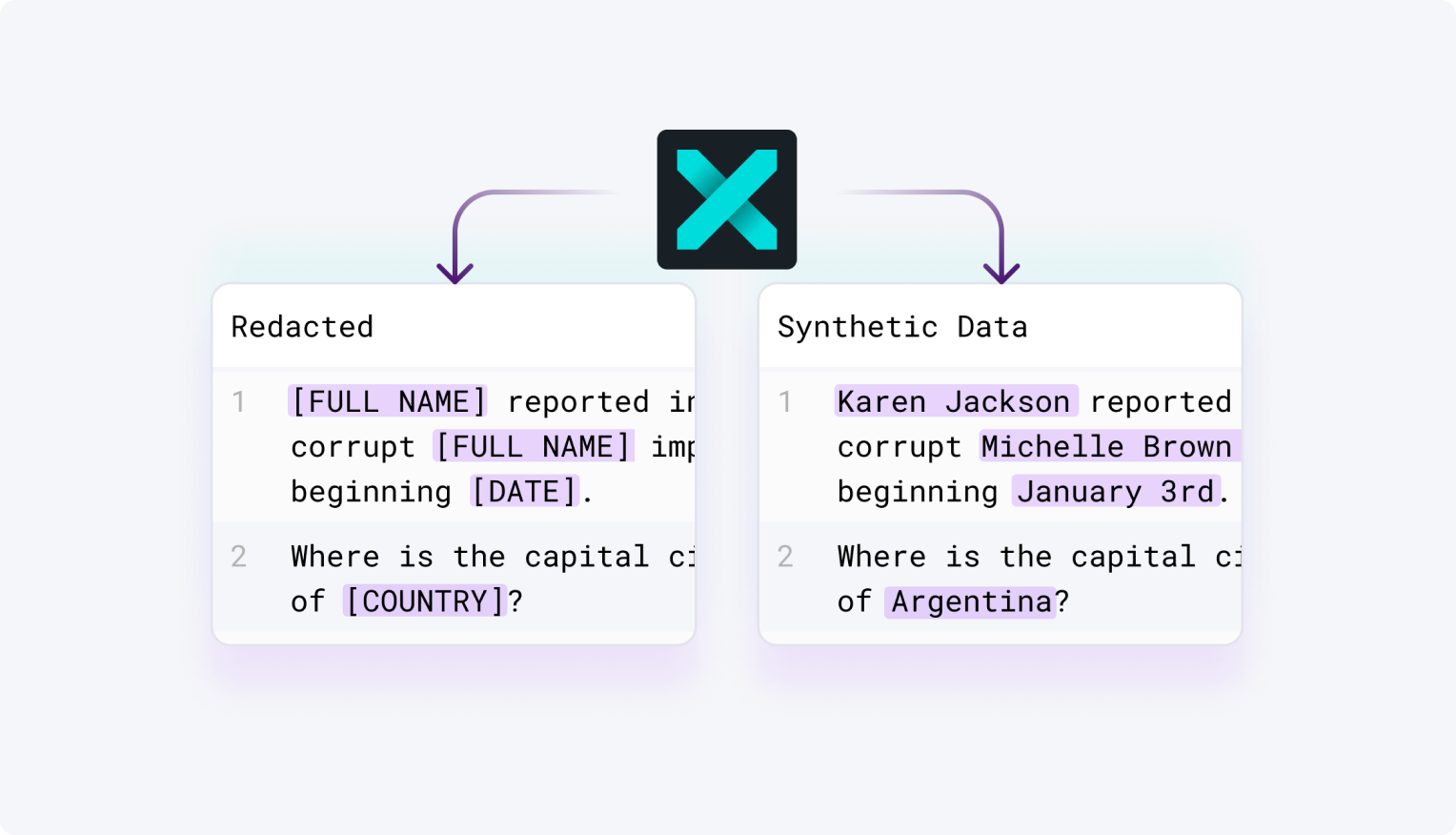Blog
Technical

# Simulating event pipelines for fun and profit (and for testing too)Author
Andrew Colombi, PhD
February 26, 2019TL/DR: You can generate events using an exponential distribution, but modeling a real world event sequence is usually more complicated than that. Here is an approach using a sequence of exponential distributions that are fit to the data using an adaptive process. And we have an implementation in Python here.

At Tonic we develop tools for data privacy, and an important tool for keeping data private is synthetic data. In this post we’ll talk about what we learned developing an algorithm for creating realistic event data. The algorithm below is one of many that make up Tonic’s data synthesis technology. Data created this way has many uses: from testing and development to sales demos and creating data partnerships. Simulated events can be fed to a streaming data pipeline (e.g. data in flight), or loaded directly into a data store (e.g. data at rest).

To start let’s agree on what “event data” is. Event data represents a sequence of things that happened; for example, patients being examined by a doctor. Common attributes found in event data include:

• the timestamp of the event,
• an entity or entities that the event pertain to, for example the patient, and the doctor,
• the state of the entities at the moment of the event, for example the patient’s diagnosis.

Event data shows up in lots of contexts: web traffic, financial transactions, health care data, to name just a few.

Our approach to data synthesis of events splits the problem into two subproblems: generating realistic timestamps and generating realistic state changes. In this blog post we’ll focus on generating realistic timestamps, and talk about state changes in a future post.

## The Exponential Distribution

Statistical modeling of event times is a well researched field of Operations Research. The main tool we’ll use is the exponential distribution. In short, the exponential distribution is a probability distribution that measures the time between two events. The exponential distribution takes one parameter, called the rate parameter and often written as $λ$ (the greek letter lambda). Given $λ$ you can create events spaced according to $λ$ with the following formula:

$X_i = -ln(U) / λ$, where $U$ is a uniformly distributed random variable between 0 and 1. $X_i$ are the times between subsequent events, i.e. they’re offsets from the previous event. Therefore, to create a timeline from $X_i$ you need to take a rolling cumulative sum of $X_i$.

Great! It sounds like all we need to do to get good event timestamps is pick a good $λ$ for our event data, pump out a bunch of $X_i$, and take a rolling cumulative sum of the result. It turns out there will be some problems with this approach, but for now let’s assume it’ll work and finish this example. The next step then, is to pick $λ$.

The simplest approach for fitting this parameter is called Maximum Likelihood Estimation (MLE). MLE seeks the value of $λ$ that would maximize the likelihood of the observed data. In other words, there is some value of $λ$ out there that makes the observed data most likely to have happened, and MLE computes it. Okay, if that still doesn’t make sense don’t worry about it, there’s a formula on Wikipedia you can use. ;-)

$λ = (n-1) / \sum_i (x_i)$, where $n$ is the number of events and $x_i$ are the observed differences between events.

And we’re off to the races! Well, not so fast. Remember I said there would be problems. Let’s imagine applying this to credit card transactions. Each event is a unique transaction on a credit card, and we have data spanning 1 year. If we simulate events in this way you’ll find there is one major problem: the simulated transactions are equally likely to occur at 3AM as 3PM. Obviously this isn’t very realistic. This problem – event rate being dependent on time – is common in real world data. From web traffic to ER visits, the world sleeps, and thus so do the events we create.

To handle this we need to introduce a new tool, non-homogeneous $λ$, i.e. a sequence of exponential distributions whose $λ$ parameters vary with time.

## Non-homogeneous λ

Intuitively we understand that events occur at different rates at different times. To model this in our event synthesizer we can vary the rate parameter, i.e. $λ$, with time. However, varying $λ$ opens two new questions, how do we synthesize timestamps when $λ$ varies, and how do we estimate $λ$ over different time periods. It turns out the exponential distribution has a very helpful property that will provide us with a solution: the exponential distribution is memoryless.

A distribution is said to be memoryless when $P(T > t + s | T > s) = P(T > t)$. In English, it’s saying the probability of an event happening at a particular instant in time is equal throughout time, i.e. it doesn’t increase or decrease as time passes. Taking a concrete example, the likelihood a meteor hits you is equal now as it will be 10 hours from now. How can we use this to property to synthesize events and fit $λ$? To start let’s suppose we have $λ_i$ and focus on synthesizing events given $λ_i$.

Let’s take a simple example where we have two $λ_i$. $λ_p$ represents the rate of events at night (6PM to 6AM) and $λ_a$ is the rate for the day (6AM to 6PM).

We begin our synthesized event stream at 6PM, using $-ln(U) / λ_p$ and one of two things will happen, either the next event will land before or after 6AM, which is the cutoff between $λ_p$ and $λ_a$. If it lands before the cutoff we record that event and then repeat the process for the next event. If it lands after the $λ_p$ cutoff then we have to switch to the $λ_a$ rate regime. What does that mean? Perhaps an analogy will help.

Imagine our simulation as a bullet traveling down the timeline; the simulation proceeds by moving the bullet through infinitesimally small time slices. Due to memorylessness, the bullet drops events with equal probability in these time slices, in accordance to the rate parameter $λ_i$. At the moment the bullet crosses the boundary between $λ_p$ and $λ_a$ the probability of an event being dropped changes.

Now suppose I told you we accidentally simulated too much, the bullet passed 6AM and we forgot to change to $λ_a$.

What would you do? Naturally you would throw away the results after 6AM, and reset the simulation using $λ_a$. Simple fix for a simple problem.

Synthesizing using the exponential distribution is not exactly the same as our bullet on a timeline analogy. We’re not simulating tiny time periods, each with equal probability of having an event. Instead the exponential distribution tells us exactly when the next event occurs. It’s analogous to zipping the bullet ahead to just the times it drops an event. However, when the bullet zips past the boundary between $λ_p$ and $λ_a$, we can use the same correction. Throw away the results for any times past the valid domain of $λ_p$, and start the simulation fresh from the start time of $λ_a$’s domain. So the algorithm looks like this (pseudocode):

<p>CODE: https://gist.github.com/chiarabeth/e556bb4b3bcd3dd2828907119d6921ff.js</p>

Fantastic, now if only we knew how to compute $λ_p$ and $λ_a$. Memorylessness comes in handy again. A memoryless distribution ignores what’s happened in the past when predicting the future (is the name memoryless starting to make sense now?), so when fitting $λ_p$ and $λ_a$ we can ignore data outside the domains of $λ_p$ and $λ_a$. Therefore the same MLE technique as before, but applied separately to the night and day events, will work. Explicitly,

$λ_p = (n_p - 1) / \sum_{i \in night} (x_i)$,
$λ_a = (n_a - 1) / \sum_{i \in day} (x_i)$,

where $n_p$ and $n_a$ are the number of night and day events respectively.

This leaves us with one last question. How do we define the buckets? Why two buckets, one from 6PM to 6AM and one from 6AM to 6PM? Why not four, or six or one-hundred? To address this issue we’ll use an adaptive approach that subdivides time, and fits $λ_i$ to each subdivision.

The last piece of the puzzle, determining where and how many $λ_i$ we need. It’s picture time again.

In this timeline we can clearly see three regimes of event periods. How can we discover the correct boundaries? Divide and conquer! At a high level our approach is to subdivide the domain of the events into progressively smaller subdomains, until our estimate of $λ$ no longer improves by further subdivision. To estimate if $λ$ would be improved by subdividing, we tentatively subdivide and compare the new would be $λ_l$ and $λ_r$. If they’re close in value we stop subdividing. A picture will do a better job of explaining.

For practical purposes we put in a few additional guardrails in the subdivision algorithm:

• A minimum number of subdivisions to perform.
• Never subdivide if it would create a subdivision with fewer than 10 events.

## Conclusion

And that about wraps it up; these are the basics of our engine for generating realistic timestamps. This is part of the algorithm used to synthesize event data in Tonic’s data synthesis platform, which you can check out here. Or, check out this small open source project which contains an implementation of the algorithm discussed above.Andrew Colombi, PhD
Co-Founder & CTO
Andrew is the Co-founder and CTO of Tonic. An early employee at Palantir, he led the team of engineers that launched the company into the commercial sector and later began Palantir’s Foundry product. Today, he is building Tonic’s platform to engineer data that parallels the complex realities of modern data ecosystems and supply development teams with the resource-saving tools they most need.Real. Fake. Data.
Say goodbye to inefficient in-house workarounds and clunky legacy tools. The data you need is useful, realistic, safe—and accessible by way of API.
The LatestIntroducing Tonic Textual: Redact and synthesize sensitive free-text dataRAG Evaluation Series: Validating the RAG Performance of LangChain vs HaystackUnlocking Secure Data Utility: The Tonic.ai and Databricks Partnership and Integration